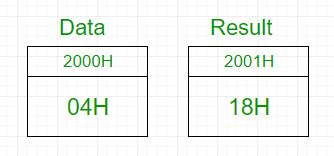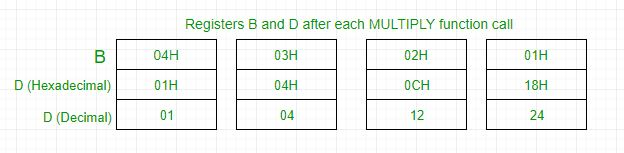# 8085 program to find the factorial of a number

• Difficulty Level : Hard
• Last Updated : 07 Sep, 2018

Problem – Write an assembly language program for calculating the factorial of a number using 8085 microprocessor.

Example –

```Input : 04H
Output : 18H
as 04*03*02*01 = 24 in decimal => 18H
```In 8085 microprocessor, no direct instruction exists to multiply two numbers, so multiplication is done by repeated addition as 4×3 is equivalent to 4+4+4 (i.e., 3 times).
Load 04H in D register -> Add 04H 3 times -> D register now contains 0CH -> Add 0CH 2 times -> D register now contains 18H -> Add 18H 1 time -> D register now contains 18H -> Output is 18HAlgorithm –

1. Load the data into register B
2. To start multiplication set D to 01H
4. Decrements B to multiply previous number
6. Take memory pointer to next location and store result
7. Load E with contents of B and clear accumulator
8. Repeatedly add contents of D to accumulator E times
9. Store accumulator content to D
10. Go to step 4
2000H Data Data Byte
2001H Result Result of factorial
2002H LXI H, 2000H Load data from memory
2005H MOV B, M Load data to B register
2006H MVI D, 01H Set D register with 1
2008H FACTORIAL CALL MULTIPLY Subroutine call for multiplication
200BH DCR B Decrement B
200CH JNZ FACTORIAL Call factorial till B becomes 0
200FH INX H Increment memory
2010H MOV M, D Store result in memory
2011H HLT Halt
2100H MULTIPLY MOV E, B Transfer contents of B to C
2101H MVI A, 00H Clear accumulator to store result
2104H DCR E Decrement E
2108H MOV D, A Transfer contents of A to D
2109H RET Return from subroutine

Explanation –

1. First set register B with data.
2. Set register D with data by calling MULTIPLY subroutine one time.
3. Decrement B and add D to itself B times by calling MULTIPLY subroutine as 4*3 is equivalent to 4+4+4 (i.e., 3 times).
4. Repeat the above step till B reaches 0 and then exit the program.
5. The result is obtained in D register which is stored in memory
My Personal Notes arrow_drop_up
Recommended Articles
Page :